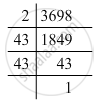Advertisement Remove all ads

# By What Numbers Should Each of the Following Be Divided to Get a Perfect Square? Also, Find the Number Whose Square is the New Number. 3698 - Mathematics

By what numbers should each of the following be divided to get a perfect square? Also, find the number whose square is the new number.

3698

Advertisement Remove all ads

#### Solution

3698 = 2 x 43 x 43Grouping them into pairs of equal factors:
3698 = 2 x (43 x 43)
The factor, 2 is not paired. For a number to be a perfect square, each prime factor has to be paired. Hence, 3698 must be divided by 2 for it to be a perfect square.
The new number would be (43 x 43).
Hence, the number whose square is the new number is 43.

Is there an error in this question or solution?
Advertisement Remove all ads

#### APPEARS IN

RD Sharma Class 8 Maths
Chapter 3 Squares and Square Roots
Exercise 3.1 | Q 8.2 | Page 4
Advertisement Remove all ads

#### Video TutorialsVIEW ALL 

Advertisement Remove all ads
Share
Notifications

View all notifications

Forgot password?
Course